# Open data might be a false good opportunity…

I am always surprised to see many people on Twitter tweeting about #opendata, e.g. @data4all, @usdatagov, @datapublicatwit, @ProPublica or @open3 among so many others… Initially, I was also very enthousiastic, but I have to admit thatopen data are rarely raw data. Which is what I am usually looking for, as a statistician…
Consider the following example: I was wondering (Valentine’s day is approaching)when will a man born in 1975 (say) get married – if he ever gets married ?More technically, I was looking for a distribution of the age of first marriage (given the year of birth), including the proportion of men that will never get married, for that specific cohort.The only data I found on the internet is the following, on statistics.gov.uk/Note that we can also focus on women (e.g. here). Is it possible to use that opendata to get an estimation of the distribution of first marriage for some specific cohort ? (and to answer the question I asked). Here, we have two dimensions: on line, the year (of the marriage), and on column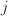, the age of the man when he gets married. Assume that those were rawdata, i.e. that we have the number of marriages of men of ageduring the year.

We are interested at a longitudinal lecture of the table, i.e. consider some man born year, we want to estimate (or predict) the age he will get married, if he gets married. With raw data, we can do it… The first step is to build up triangles (to have a cohort vs. age lecture of the data), and then to consider a model, e.g.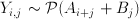where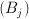is a year effect, and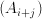is a cohort effect.

```base=read.table("http://freakonometrics.free.fr/mariage-age-uk.csv",
m=base[1:16,]
m=m[,3:10]
m=as.matrix(m)
triangle=matrix(NA,nrow(m),ncol(m))
n=ncol(m)
for(i in 1:16){
triangle[i,]=diag(m[i-1+(1:n),])
}
triangle[nrow(m),1]=m[nrow(m),1]

triangle
[,1] [,2] [,3] [,4] [,5] [,6] [,7] [,8]
[1,]   12  104  222  247  198  132   51   34
[2,]    8   89  228  257  202  102   75   49
[3,]    4   80  209  247  168  129   92   50
[4,]    4   73  196  236  181  140   88   45
[5,]    3   78  242  206  161  114   68   47
[6,]   11  150  223  199  157  105   73   39
[7,]   12  117  194  183  136   96   61   36
[8,]   11  118  202  175  122   92   62   40
[9,]   15  147  218  162  127   98   72   48
[10,]   20  185  204  171  138  112   82   NA
[11,]   31  197  240  209  172  138   NA   NA
[12,]   34  196  233  202  169   NA   NA   NA
[13,]   35  166  210  199   NA   NA   NA   NA
[14,]   26  139  210   NA   NA   NA   NA   NA
[15,]   18  104   NA   NA   NA   NA   NA   NA
[16,]   10   NA   NA   NA   NA   NA   NA   NA

Y=as.vector(triangle)
YEARS=seq(1918,1993,by=5)
AGES=seq(22,57,by=5)
X1=rep(YEARS,length(AGES))
X2=rep(AGES,each=length(YEARS))
reg=glm(Y~as.factor(X1)+as.factor(X2),family="poisson")
summary(reg)

Call:
glm(formula = Y ~ as.factor(X1) + as.factor(X2), family = "poisson")

Deviance Residuals:
Min       1Q   Median       3Q      Max
-5.4502  -1.1611  -0.0603   1.0471   4.6214

Coefficients:
Estimate Std. Error z value Pr(>|z|)
(Intercept)        2.8300461  0.0712160  39.739  < 2e-16 ***
as.factor(X1)1923  0.0099503  0.0446105   0.223 0.823497
as.factor(X1)1928 -0.0212236  0.0449605  -0.472 0.636891
as.factor(X1)1933 -0.0377019  0.0451489  -0.835 0.403686
as.factor(X1)1938 -0.0844692  0.0456962  -1.848 0.064531 .
as.factor(X1)1943 -0.0439519  0.0452209  -0.972 0.331082
as.factor(X1)1948 -0.1803236  0.0468786  -3.847 0.000120 ***
as.factor(X1)1953 -0.1960149  0.0470802  -4.163 3.14e-05 ***
as.factor(X1)1958 -0.1199103  0.0461237  -2.600 0.009329 **
as.factor(X1)1963 -0.0446620  0.0458508  -0.974 0.330020
as.factor(X1)1968  0.1192561  0.0450437   2.648 0.008107 **
as.factor(X1)1973  0.0985671  0.0472460   2.086 0.036956 *
as.factor(X1)1978  0.0356199  0.0520094   0.685 0.493423
as.factor(X1)1983  0.0004365  0.0617191   0.007 0.994357
as.factor(X1)1988 -0.2191428  0.0981189  -2.233 0.025520 *
as.factor(X1)1993 -0.5274610  0.3241477  -1.627 0.103689
as.factor(X2)27    2.0748202  0.0679193  30.548  < 2e-16 ***
as.factor(X2)32    2.5768802  0.0667480  38.606  < 2e-16 ***
as.factor(X2)37    2.5350787  0.0671736  37.739  < 2e-16 ***
as.factor(X2)42    2.2883203  0.0683441  33.482  < 2e-16 ***
as.factor(X2)47    1.9601540  0.0704276  27.832  < 2e-16 ***
as.factor(X2)52    1.5216903  0.0745623  20.408  < 2e-16 ***
as.factor(X2)57    1.0060665  0.0822708  12.229  < 2e-16 ***
---
Signif. codes:  0 ‘***’ 0.001 ‘**’ 0.01 ‘*’ 0.05 ‘.’ 0.1 ‘ ’ 1

(Dispersion parameter for poisson family taken to be 1)

Null deviance: 5299.30  on 99  degrees of freedom
Residual deviance:  375.53  on 77  degrees of freedom
(28 observations deleted due to missingness)
AIC: 1052.1

Number of Fisher Scoring iterations: 5```

Here, we have been able to derive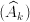and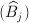, where now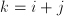denotes the cohort.
We can now predict the number of marriages per year, and per cohortHere, given the cohort, the shape of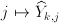is the following

```Yp=predict(reg,type="response")
tYp=matrix(Yp,nrow(m),ncol(m))
tYp[16,]
tYp[16,]
  10.00000 222.94525 209.32773 159.87855 115.06971  42.59102
  18.70168 148.92360```The errors (Pearson error) look like that
`Ep=residuals(reg,type="pearson")`(where the darker the blue, the smaller the residuals, and the darker the red, the higher the residuals). Obviously, we are missing something here, like a diagonal effect. But this is not the main problem here…I guess that study here is not valid. The problem is that we deal with open data, and numbers of marriages are not given here: what is given is a he proportion of marriage of men of ageduring the year, with a yearly normalization. There is a constraint on lines, i.e. we observe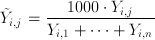so that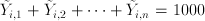This is mentioned in the titleIt is still possible to consider a Poisson regression on the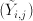, but unfortunately, I do not think any interpretation is valid (unless demography did not change last century). For instance, the following sum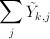looks like that

```apply(tYp,1,sum)
 919.948 838.762 846.301 816.552 943.559 930.280 857.871 896.113
 905.086 948.087 895.862 853.738 826.003 816.192 813.974 927.437```

i.e. if we look at the graphBut I do not think we can interpret that sum as the probability (if we divide by 1,000) that a man in that cohort gets married…. And more basically, I cannot do anything with that dataset…So open data might be interesting. The problem is that most of the time, the data are somehow normalized (or aggregated). And then, it becomes difficult to use them…

So I will have to work further to be able to write something (mathematically valid) on marriage strategy before Valentine’s day…. to be continued.

## 2 thoughts on “Open data might be a false good opportunity…”

1.W David Stephenson says:

Couldn’t agree more that aggregated or normalized data is worthless. IMHO, the Holy Grail is tagging data THE FIRST TIME (sorry for shouting, but I really believe this) it is entered anywhere in the organization, and releasing that tagged data. The places it can be used internally and externally will vastly increase, and we will switch from talking about data warehousing to “where else could this data produce value?”

This site uses Akismet to reduce spam. Learn how your comment data is processed.